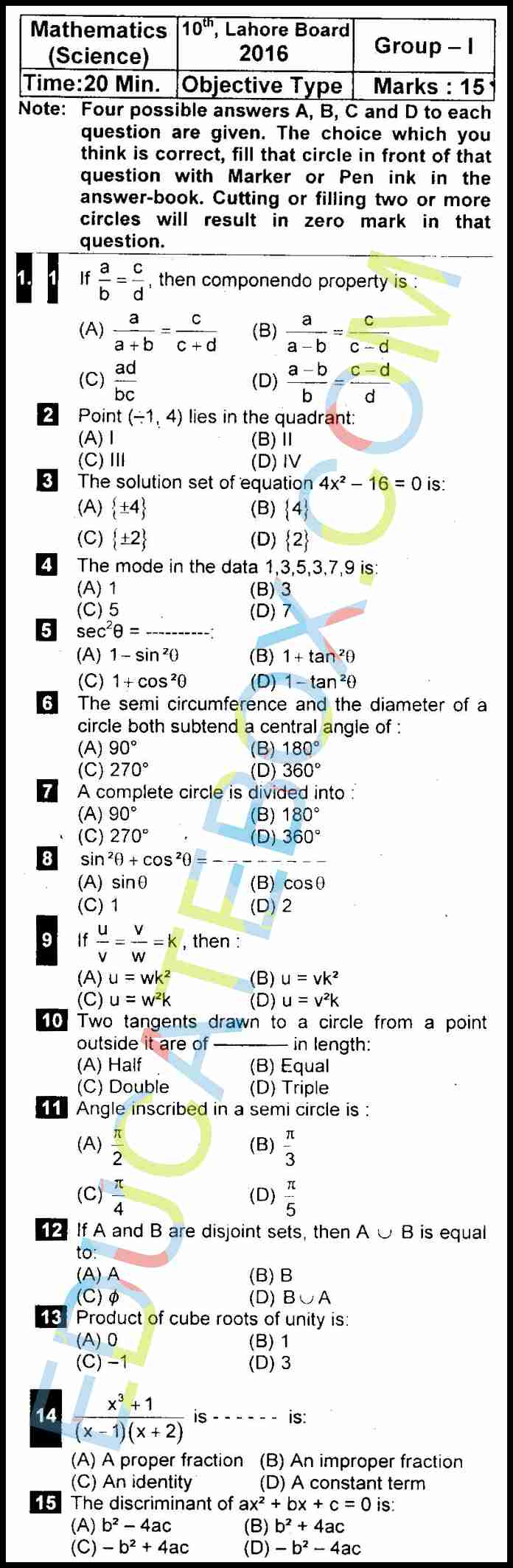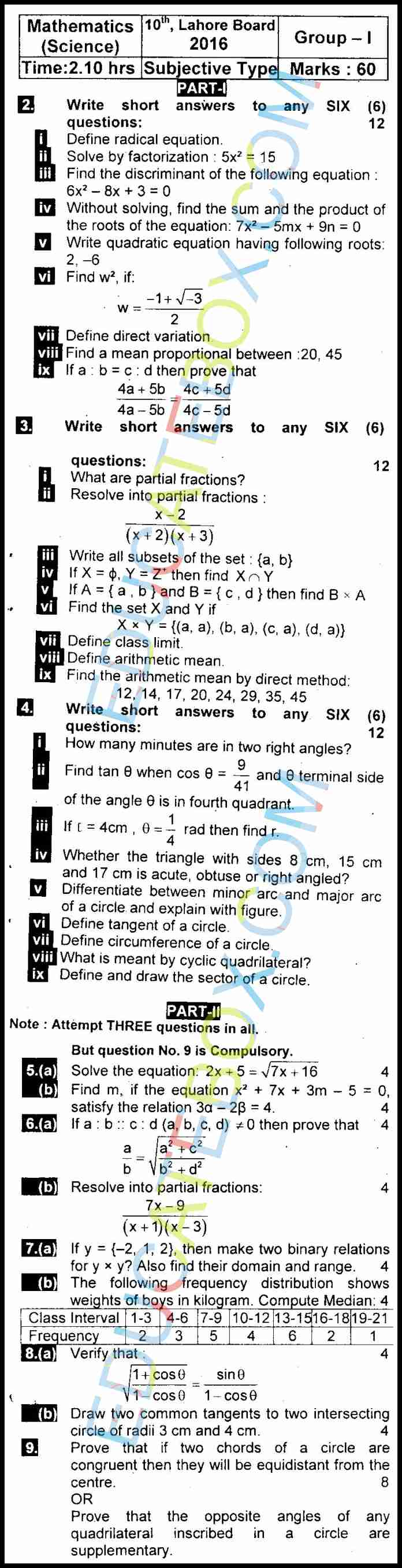# Past Paper 10th Maths Lahore 2016 Group 1

0
237

## Past Paper Class 10 Maths Lahore Board 2016 Objective Type Group 1 (English Medium)

Here is 2016, Group 1 past paper of Maths for 10th class. It is English medium version of this Maths paper. The objective part contains 15 MCQs. The time allowed is fifteen minutes for this part of paper. First three questions and ninth questions are relating to equations. There are some geometry questions and some other about mean, median etc.## Past Paper Class 10 Maths Lahore Board 2016 SUBJECTIVE Type Group 1 (English Medium)

The subjective is a 60 marks paper with combination of short and long questions. The questions in this part include equation, factorization, quadratic equation, arithmetic mean, fraction, subset, angles, circles, and more.